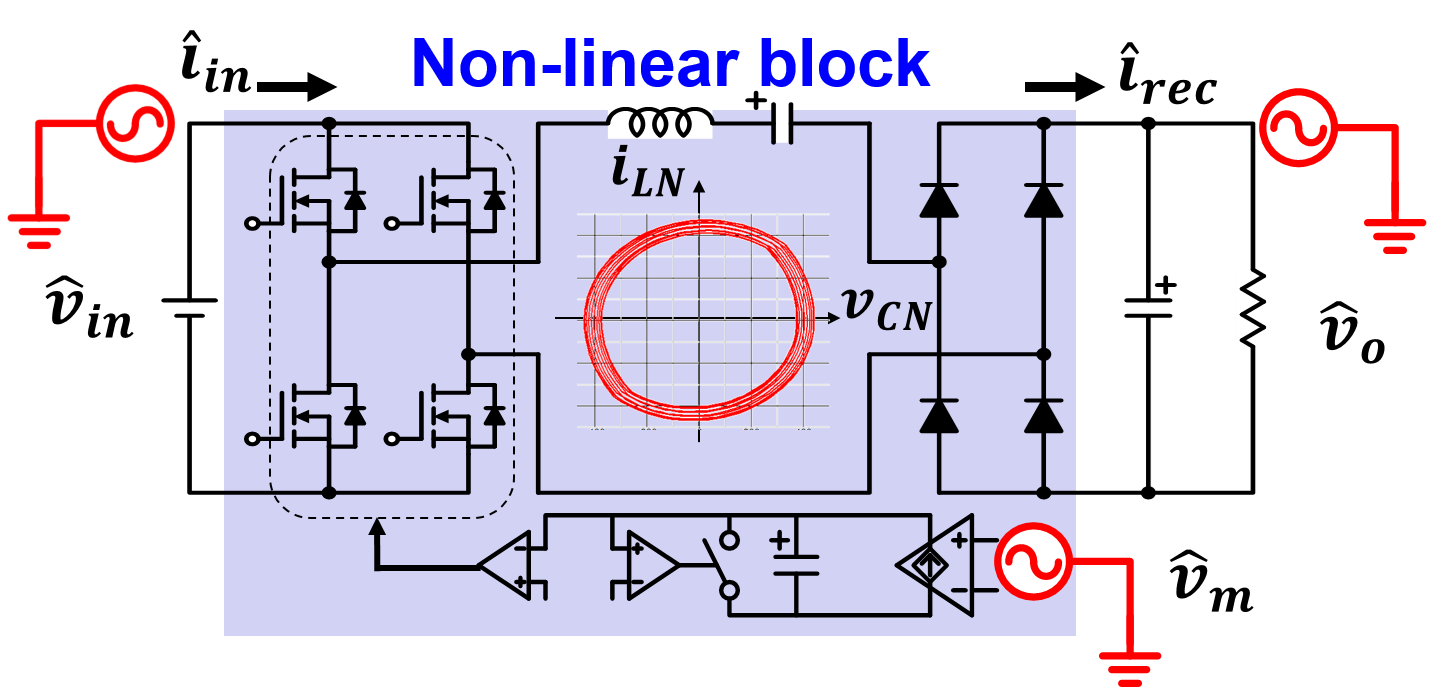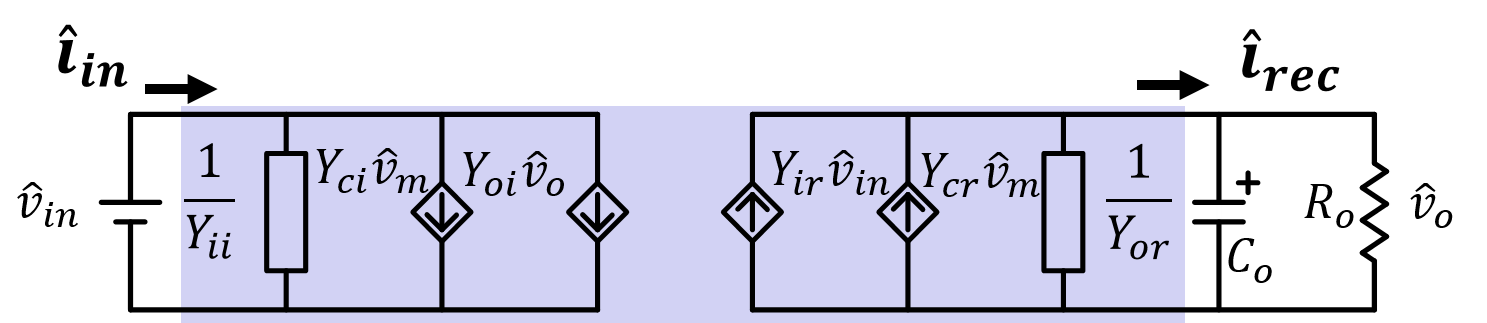Contact Us
LIBRARY

# Accurate Small-Signal Equivalent Circuit Model for Resonant ConvertersFig.1. Perturbation to a series resonant converter
Resonant converters, as shown in Fig. 1, are the preferred topologies of a wide range of applica-tions. The major benefits of resonant converters, especially for LLC converters, are zero-voltage switching (ZVS) from zero to full load, low turn-off current of primary-side devices, zero-current switching (ZCS) of rectifiers, and circuit simplicity. Therefore, a power converter is made efficient and compact. Despite decades of popularity, no simple and accurate small-signal model is available. One modeling approach is the discrete-time model. However, the result is overly complicated and often numerically solved. Besides, there is no extension to an LLC converter using this method. The other approach is based on fundamental approximation. Using this method, E. Yang developed an equivalent circuit model for series resonant converters (SRC). His modeling method was extended to LLC converters by C. Chang and S. Tian. Still complicated, the equivalent circuit model was is then simplified by S. Tian. However, the major assumption for the fundamental approximation is the resonant tank operates as a good band-pass filter, which is normally not the case especially for LLC converters. As a result, the existing small-signal models work to a certain extent for SRCs but not LLC converters. In this paper, a new modeling approach based on an extended describing function method is proposed. Referring to Fig. 1, the non-linear part of the resonant converter, which consists of an inverter, resonant tank, rectifier, and voltage-controlled oscillator (VCO), is first identified. Using superposition theory, the describing functions of the non-linear part subject to control, input voltage, and output voltage excitations are derived. Combining the non-linear part to the rest of the linear circuit, a simple equivalent circuit model is proposed and shown in Fig. 2. Without any assumption, the proposed small-signal model is accurate beyond switching frequency. The proposed equivalent circuit model indicates, not surprisingly, a resonant converter is an infi-nite-pole system. For controller design and dynamic analysis, the frequency of interest is lower than the switching frequency in most cases. Ignoring high frequency poles, a simplified equivalent circuit model is proposed and as shown in Fig 3. The small-signal behavior is well-explained with the simplified equivalent circuit model, including the beat-frequency double pole (from resonance between Le and Ce) and the energy stored in the resonant tank (Le). Furthermore, the right-half-plane (RHP) zero (caused by negative Rz) is predicted and explained for the first time for resonant converters. The prediction of the RHP zero is a significant contribution for an optimal controller design.Fig. 2. Proposed small-signal model.
,Fig. 3. Simplified small-signal equivalent circuit model observed from output side
INDUSTRY PARTNERS